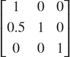Apply Horizontal Shear Transformation to Image

Read an image into the MATLAB workspace.

Display the input image.

figure
imshow(I)
title('Input Image')modelname = 'ex_shearblkwarp.slx';
open_system(modelname)The model reads the input image from the workspace by using the Image From Workspace block. To apply horizontal shear transformation, you must specify the transformation matrix at the input by using TForm input port.

The model then shears the image to the horizontal direction by using the Warp block with these parameter values:

• Transformation matrix source - Input port. This enables the TForm input port. Specify the horizontal shear transformation matrix value asby using the Constant block.

• Interpolation method - Bilinear

• Background fill value - 0

• Output image position source - Custom

• Output image position vector [x y width height] - [-20 -20 740 430]

Run the model and display the output transformed image by using the Video Viewer block.

sim(modelname);